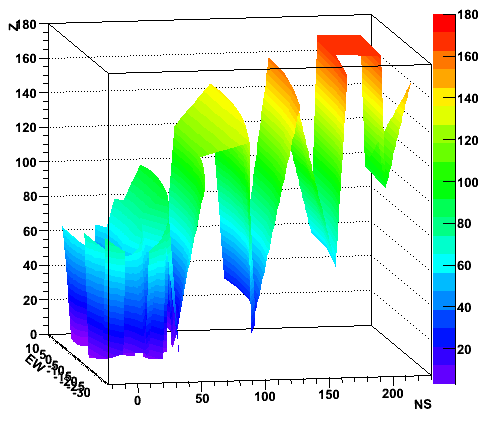# TGraph3D?

Hi,

I’m trying to plot a surface in 3 dimensions, ideally with a fourth coordinate plotted as the color.

A TGraph2D “fails” when two or more surfaces are on the same xy-area: the Delaunay triangles are calculated in the xy plane and and lead to just one distorted layer (cf plot).

Is there something like a TGraph3D to do what I want simply (ie plot a surface between a set of points - eventually giving explicitly which points should be linked together - and drawing this surface with a color given by a fourth coordinate)?

Or should I do it by hand using just the geometry package and define many triangles? Any better solution?

Thanks,
ColasThere is not easy answer. I thought the GL tool we have might help but we had an mail exchange Timur (who implemented them) and there is nothing like that available right now. The only 3D plot are the density plots. So yes your initial idea might be the solution.

If your original data are in a Tree, use the 4-D capabilities of TTree::Draw
to make a 4-D scatter-plot where you assign the 4th variable to teh color

Rene